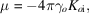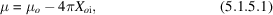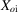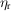International
Tables for
Crystallography
Volume B
Reciprocal space
Edited by U. Shmueli

International Tables for Crystallography (2010). Vol. B, ch. 5.1, pp. 633-634   | 1 | 2 |

## Section 5.1.5. Anomalous absorption

A. Authiera*

aInstitut de Minéralogie et de la Physique des Milieux Condensés, Bâtiment 7, 140 rue de Lourmel, 75015 Paris, France

### 5.1.5. Anomalous absorption

| top | pdf |

It was shown in Section 5.1.2.2that the wavevectors of a given wavefield all have the same imaginary part (5.1.2.17)and therefore the same absorption coefficient μ (5.1.2.19). Borrmann (1950, 1954) showed that this coefficient is much smaller than the normal onefor wavefields whose tie points lie on branch 1 of the dispersion surface and much larger for wavefields whose tie points lie on branch 2. The former case corresponds to the anomalous transmission effect, or Borrmann effect. As in favourable cases the minimum absorption coefficient may be as low as a few per cent of, this effect is very important from both a fundamental and a practical point of view.

The physical interpretation of the Borrmann effect is to be found in the standing waves described in Section 5.1.4. When the nodes of the electric field lie on the planes corresponding to the maxima of the hkl component of the electron density, the wavefields are absorbed anomalously less than when there is no diffraction. Just the opposite occurs for branch 2 wavefields, whose anti-nodes lie on the maxima of the electron density and which are absorbed more than normal.

The effective absorption coefficient μ is related to the imaginary part of the wavevectors through (5.1.2.19),and to the imaginary part of the ratio of the amplitude of the reflected to the incident wave throughwhereis the imaginary part of, which, using (5.1.2.24)and (5.1.3.10), is given byTaking the upper sign (+) for the ± term corresponds to tie points on branch 1 and taking the lower sign (−) corresponds to tie points on branch 2.

The calculation of the imaginary partis different in the Laue and in the Bragg cases. In the former case, the imaginary part ofis small and can be approximated while in the latter, the imaginary part ofis large when the real part of the deviation parameter,, lies between 1 and −1, and cannot be calculated using the same approximation.

### References

Borrmann, G. (1950). Die Absorption von Röntgenstrahlen in Fall der Interferenz. Z. Phys. 127, 297–323.
Borrmann, G. (1954). Der kleinste Absorption Koeffizient interfierender Röntgenstrahlung. Z. Kristallogr. 106, 109–121.